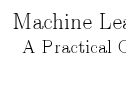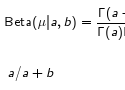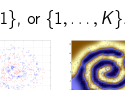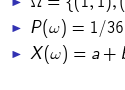# Teaching

I've set up a google group where you can get latest information on the current teaching activities, ask questions, and discuss issues. You have to become a member to view the group contents, but access is not restricted.

## Courses

### Winter Term 2007/2008

During the winter term 2007/2008 I was on parental leave.

## Teaching Material### Machine Learning—A Practical Course

This script has been written by Paul Bünau and me as supplementary material to the lab course on machine learning. In it we try to follow a "hands-down" approach to machine learning. For each method, you find a categorization, a brief explanation of how the method works, and, most importantly, pseudo code to implement the method quickly.### Some Introductory Remarks on Bayesian Inference

This talk gives introduces the basic ideas behind Bayesian inference (that is, Bayes rule). The concept of conjugate prior is discussed on some simple distributions, including how to "guess" the conjugate prior to some distribution. Finally, some more high-level discussion of the differences between Bayesian and frequentist approaches are discussed.

Disclaimer: I'm not a Bayesian `:)`### Introduction to Machine Learning

This talk gives a short overview over machine learning, including typical applications (well, mostly applications from our group).### Concepts of Probability Theory for Machine Learning

This is actually something I've been thinking about for some time. I always thought that having only a "naive" understanding of probability theory becomes a bit limiting at some point and that it would be helpful if you had seen at least once how probability theory is "built" in mathematics. Therefore, in this talk I try to introduce all the normal concepts but also give some idea of what that means mathematically.